# Scala入门

30 Mar 2021

## Scala基础

### Scala语言特点

Scala是一门以 JVM 为运行环境并将面向对象和函数式编程的最佳特性结合在一起的静态类型编程语

Scala源代码会被编译成Java字节码，然后运行于JVM之上，并可以调用现有的Java类库，实现两种语言 的无缝互操作的。

• 面向对象 Scala是一种面向对象的语言。 Scala中的每个值都是一个对象，包括基本数据类型(即布尔值、数字等)在内，连函数也是对象。

• 函数式编程 Scala也是一种函数式语言，其函数也能当成值来使用。 Scala中支持高阶函数，允许嵌套多层函数，并支持柯里化。
Scala提供了模式匹配，可以匹配各种情况，比如变量的类型、集合的元素、有值或无值。

• 静态类型 Scala具备类型系统，通过编译时检查，保证代码的安全性和一致性。

• 并发性 Scala使用Actor作为其并发模型，Actor是类似线程的实体。 Actor可以复用线程，因此可以在程序中使用数百万个Actor，而线程只能创建数千个。

### 基础语法

• 区分大小写 - Scala语言对大小写敏感;
• 类名 - 对于所有的类名的第一个字母要大写。如果需要使用几个单词来构成一个类名，每个单词的
第一个字母要大写;比如:ListDemo
• 方法名 - 所有方法名的第一个字母用小写。如果需要使用几个单词来构成方法名，除第一个单词外每个词的第一个字母应大写;比如:getResult
• 程序文件名 - Scala程序文件的后缀名是 .scala，程序文件的名称可以不与对象名称完全匹配。这点与Java有所区别。
备注:建议遵循 Java 的惯例，程序文件名称与对象名称匹配;
• main()方法 - Scala程序从main()方法开始处理，这是每一个Scala程序的入口点。main()定义在 object中;
• 标识符。所有Scala组件都需要名称，用于对象、类、变量和方法的名称称为标识符。 关键字不能用作标识符，标识符区分大小写; 标识符以字母或下划线开头，后面可以有更多的字母、数字或下划线; \$字符是Scala中的保留关键字，不能在标识符中使用;
• 注释。Scala使用了与Java相同的单行和多行注释;
• 换行符。Scala语句可以用分号作为一行的结束，语句末尾的分号通常可以省略，但是如果一行里有多
个语句那么分号是必须的。

### 常用类型与字面量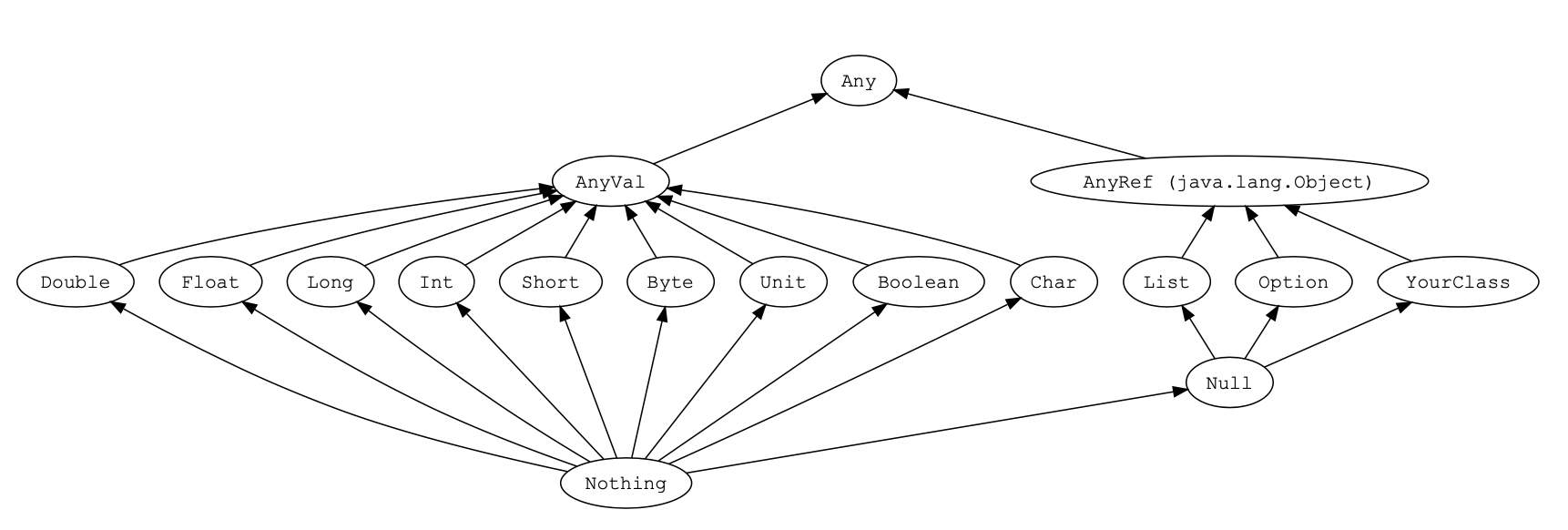Scala和Java一样，有8种数值类型 Byte、Short、Int、Long、Float、Double、Char、Boolean 类型;

Scala并不刻意的区分基本类型和引用类型。
String 直接引用 Java.lang.String 中的类型，String在需要时能隐式转换为StringOps，因此不需要任何 额外的转换，String就可以使用StringOps中的方法。

Scala中，所有的类，包括值类型和引用类型，都最终继承自一个统一的根类型Any。 Scala中定义了以下三个底层类:

• Any是所有类型共同的根类型，Any是AnyRef和AnyVal的超类
• AnyRef是所有引用类型的超类
• AnyVal是所有值类型的超类
• Null是所有引用类型的子类型
Null类只有一个实例对象null。 null可以赋值给任意引用类型，但是不能赋值给值类型。
• Nothing位于Scala类继承关系的底部，它是其他所有其他类型的子类型
• Nothing对泛型结构有用 。比如，空列表Nil的类型就是List[Nothing]
• Nothing的可以给出非正常终止的信号。比如，使用Nothing处理异常
• Unit类型用来标识过程，过程就是没有返回值的方法，Unit类似于Java里的void。Unit只有一个实 例()。

### 值与变量&自动类型推断

Scala当中的声明变量可以使用以下两种方式:

• val，值 – value，用val定义的变量，值是不可变的
• var，变量 – variable，用var定义的变量，值是可变的
在Scala中，鼓励使用val。大多数程序并不需要那么多的var变量。 声明变量时，可以不指定变量的数据类型，编译器会根据赋值内容自动推断当前变量的数据类型。
备注:简单数据类型可以省略，对于复杂的数据类型建议明确声明;
声明变量时，可以将多个变量放在一起声明。
``````-- val定义的变量不可更改，变量的类型编译器可以进行自动类型推断
val name = "zhangsan"
-- 必要时可以指定数据类型
var name: String = null
-- 可以将多个值或变量放在一起声明
val x, y = 100;
var name, message: String = null
``````

### 操作符

Scala的算术操作符、位操作符与 Java中的效果一样的。

``````a + b 等价 a.+(b)
1 to 10 等价 1.to(10)
``````

Scala 没有提供 ++、– 操作符，但是可以使用+=、-=

### 块表达式和赋值语句

{} 块包含一系列表达式，其结果也是一个表达式，块中最后一个表达式的值就是块的值。

``````val x1 = 1
val y1 = 1
val x2 = 0
val y2 = 0
val distance = {
val dx = x1 - x2
val dy = y1 - y2
math.sqrt(dx*dx + dy*dy)
}
-- 赋值语句的值是Unit类型，不要把它们串接在一起。x的值是什么?
var y = 0
val x = y = 1

// x:Unit = ()
``````

### 输入和输出

print、println、printf 可以将结果输出到屏幕;

``````print("Please input your name: ")

println("name:" + name + ", age:" + age)

println(s"name \$name, age : \$age")

printf("name: %s, age: %d", name, age)

/**
* name:APRIL, age:189
* name APRIL, age : 189
* name: APRIL, age: 189
*/
``````

### 字符串插值器

Scala 提供了三种字符串插值器:

• s 插值器，对内嵌的每个表达式求值，对求值结果调用toString，替换掉字面量中的那些表达式
• f 插值器，它除s插值器的功能外，还能进行格式化输出，在变量后用%指定输出格式，使用 java.util.Formatter中给出的语法
• raw 插值器，按照字符串原样进行输出
``````//s 插值器，对内嵌的每个表达式求值，对求值结果调用toString，替换掉字面量中的那些表达式
val subject = "Scala"
val message = s"Hello, \$subject"
println(message)

val arr = (1 to 10).toArray
val str2 = s"arr.length = \${arr.length}"
println(str2)
println(s"9 * 9 = \${9 * 9}")
/**
* Hello, Scala
* arr.length = 10
* 9 * 9 = 81
*/

//f 插值器，它除s插值器的功能外，还能进行格式化输出，在变量后用%指定输出格式，使用 java.util.Formatter中给出的语法
val year = 2021
val month = 8
val day = 15
println(s"\$year - \$month%02d - \$day")
println(f"\$year - \$month%02d - \$day")
/**
* 2021 - 8%02d - 15
* 2021 - 08 - 15
*/

//raw 插值器，按照字符串原样进行输出
println("a\nb\tc")
println(raw"a\nb\tc")
println("""a\nb\tc""")
println(
"""
|a\nb\tc
|hello world
|this is a message from the
|universe
|""".stripMargin)
``````

### 对象相等性

Java 中可以 == 来比较基本类型和引用类型:

• 对基本类型而言，比较的是值的相等性
• 对引用类型而言，比较的是引用相等性，即两个变量是否指向JVM堆上的同个对象
``````println(1==1) //true
println(1!=2)  //true
println(1==2)   //false

val flag = List(1,2,3)==List(4, 5, 6)
println(flag)  //false

println(List(1, 2, 3) != Array(1, 2, 3))  //true

println(2==2.0)  //true

println(List(1,2,3)=="Scala")  //false
``````

## 控制结构和函数

### if 表达式

``````val num=20

// if else 有返回值, 同python
// alt + enter 自动添加数据类型
val name: String = if (num > 20) "kaclyu" else "lisi"
println(name)

// 如果返回的类型不一致就返回公共的父类
val name2: Any = if (num > 20) "kaclyu" else 30
println(name2)

//  缺省 else 语句;s1/s2的返回类型不同
val s1 = if (x > 0) 1 等价 val s1 = if (x > 0) 1 else ()
val s2 = if (x > 0) "positive" 等价  val s2 = if (x > 0) "positive" else ()
``````

### for 表达式

Scala中，for循环语法结构:for (i <- 表达式 / 集合)，让变量 i遍历<-右边的表达式/集合的所有值。
Scala为for循环提供了很多的特性，这些特性被称之为 for守卫式 或 for推导式。

`````` for (i <- 1 to 10) {
//      println(i)
}

for (i <- 1 until 10) {
//      println(i)
}

//多重循环。条件之间使用分号分隔
/**
* 从右往左，一层层遍历, 每次添加一个 ； 就加一层
*/
for (i <- 1 to 4; j <- 2 to 10) {
//      println(i, j)
}
for (i <- 1 to 4; j <- 2 to 5; k <- 1 to 3) {
//            println(i, j, k)
}

// 使用变量
for (i <- 1 to 3; j=4-i) {
//      println(i, j)
}

//    守卫语句。增加 if 条件语句, 添加过滤条件，只遍历满足条件的值
for (i <- 1 to 10; j <- 3 to 5 if i==j) {
//      println(i, j)
}

// 使用 yield 接收返回的结果，这种形式被称为for推导式
// yield中的处理，留待后面使用, 相当于map处理
val result: IndexedSeq[Int] = for (i <- 1 to 10) yield i*2
val result2: IndexedSeq[Int] = (1 to 10).map(_*2)
//    result.foreach(println(_))
//    result2.foreach(println(_))

// 使用大括号将生成器、守卫、定义包含在其中;并以换行的方式来隔开它们
println("======================九九乘法表==========================")
for {i <- 1 to 9
j <- 1 to i} {
printf(s"\$i * \$j = \${i*j}\t")
if (i==j) println()
}

val message = "sparkscala"
for (c <- message) print(c + " ")
``````

### while 表达式

Scala提供了与 Java 类似的while和do…while循环。while语句的本身没有任何返回值类型，即while语

• 使用Boolean类型的控制变量
• 使用return关键字
• 使用breakable和break，需要导入scala.util.control.Breaks包
``````var num=1
while (num < 10) {
println(s"num = \$num")
num += 1
}

do {
println(s"num = \$num")
num += 1
} while (num < 10)

var flag = true
while (flag) {
println(num)
num+=1
if (num==10) flag = false
}

for (i <- 1 to 10) {
if (i==5) return
println(i)
}

import scala.util.control.Breaks._

var res = 0
breakable{
for (i <- 1 to 10) {
if (i == 5) break
res += i
}
}

println(res)
``````

### 函数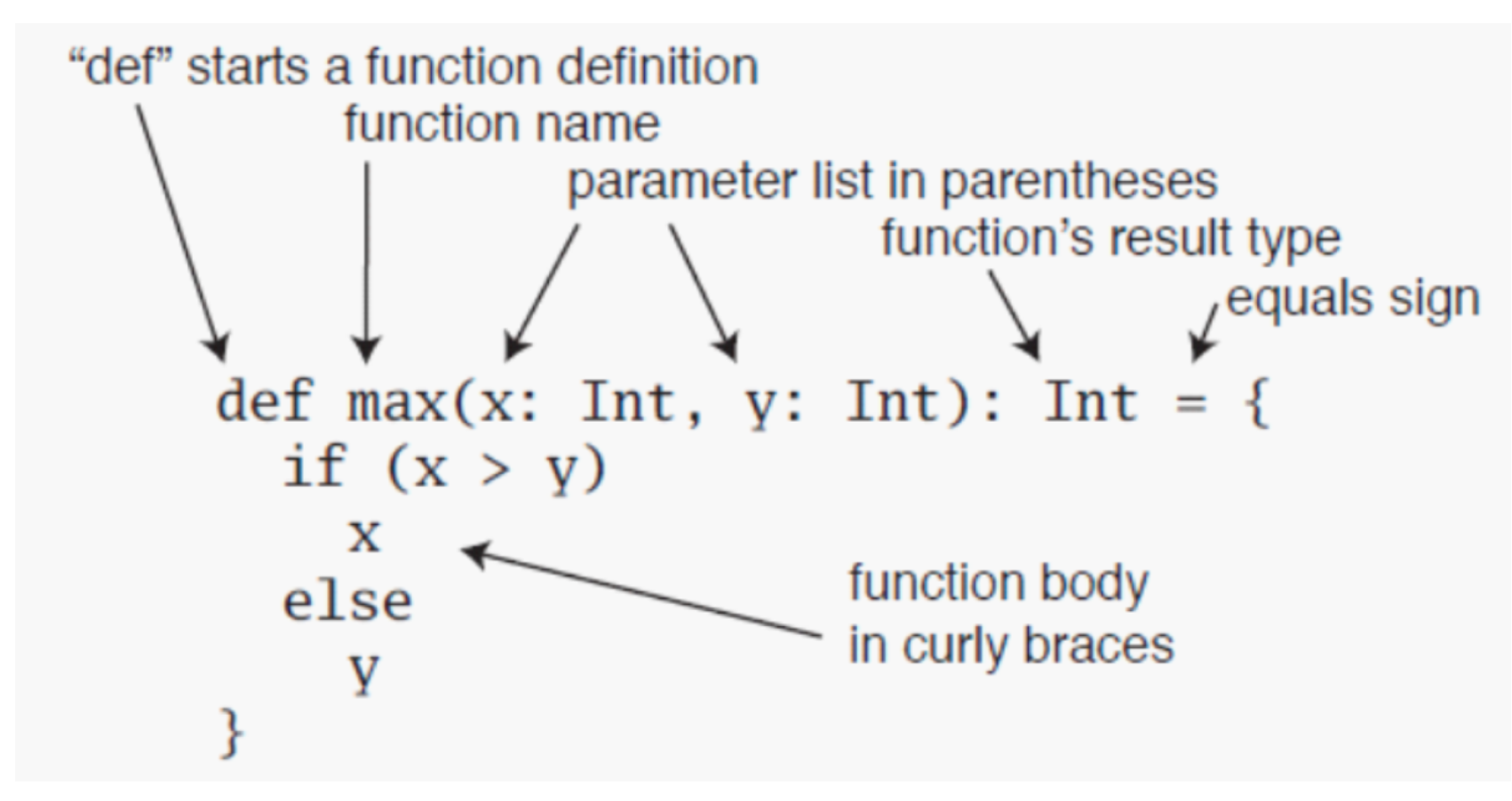• 函数体中最后一句为返回值的话，可以将return 去掉; 如果一个函数体只有一句代码，大括号可以去掉;
• 如果一个函数没有返回值，其返回类型为Unit , 并且 “=” 号可以去掉，这样的函数被称为过程;
• 可以不声明函数的返回类型，返回类型可通过自动类型推断来完成，但递归函数的返回类型必须声明;
• 建议明确声明函数的返回值，即使为Unit
``````object FuctionDemo {

/**
* 函数体中最后一句为返回值的话，可以将return 去掉
* @param num1
* @param num2
* @return
*/
def add(num1: Int, num2: Int)  = {
num1+num2
}

// 递归函数的返回类型必须声明
def facorial(num: Int): Long = {
if (num <= 1) 1 else num * facorial(num - 1)
}

def fibonacci(n:Int):Long={
if (n <= 2) 1 else fibonacci(n-1) + fibonacci(n-2)
}

// 如果一个函数没有返回值，其返回类型为Unit , 并且 “=” 号可以去掉，这样的函数被称为过程;
//  def getSum(x:Int, y:Int) = println(x+y)
def getSum(x:Int, y:Int) {
println(x+y)
}

def add2(num1: Int=10, num2: Int=20)  = {
num1+num2
}

//  变长参数只能出现在参数列表的尾部，只能有一个
nums.sum
}

def main(args: Array[String]): Unit = {

println(facorial(3))

println(fibonacci(6))

getSum(2,4)

// 缺省赋值，按顺序传递
// 指定需要赋值的key，不需要按顺序传递

//告诉编译器这个参数被当做参数序列处理。使用 parameter: _* 的形式
}

}

``````

### 懒值

``````// 文件不存在时，不会报错。因为语句此时并没有被执行
lazy val file1 = scala.io.Source.fromFile("src/test.scala")

println("Okay")

// 先打印OK!，才报错
println(file1.getLines().size)
``````

### 文件操作

• 相比于java中的IO类，Scala的IO类较少，最常用的是Source这个类。更多的是调用java中的IO类，或对java类进行二次封装。
• 导入scala.io.Source后，可引用Source中的方法读取文本文件的内容
• Scala没有内建的对写入文件的支持。要写入文本文件，可使用 java.io.PrintWriter
``````def readTextFile:Unit = {
val file: BufferedSource = scala.io.Source.fromFile("src/text.txt")
val lines: Iterator[String] = file.getLines()
lines.foreach(println(_))
file.close()
}

val source: BufferedSource = scala.io.Source.fromURL("https://www.baidu.com")
println("source.codec " + source.codec)
println(source.mkString)

source.close()
}

//Scala没有内建的对写入文件的支持。要写入文本文件，可使用 java.io.PrintWriter

def writeTextFile: Unit = {
val writer = new PrintWriter("src/text.txt")

(1 to 10).foreach(writer.println(_))
writer.flush()

writer.close()
}

def main(args: Array[String]): Unit = {
writeTextFile
}
``````

## 数组和元组

### 数组定义

• 定长数组
``````// 定长数组 ，Array
//    -- 长度为10的整型数组，初始值为0
val nums = new Array[Int](10)
// -- 省略new关键字，定义数组，scala进行自动类型推断
val strs = new Array[String](10)

//    -- 使用()访问数据元素;下标从0开始
println(nums(2))
println(strs(3))

// -- 省略new关键字，定义数组，scala进行自动类型推断
val ints: Array[Int] = Array(1, 2, 3)
println(ints.apply(2))

val array: Array[Nothing] = Array()

//    -- 快速定义数组，用于测试
val array1: Array[Int] = (1 to 10).toArray
``````
• 变长数组

``````/**
* 变长数组
* 长度按需要变换的数组ArrayBuffer。Scala 中很多数组类型都有可变、不可变两个版本，推荐使用不可
* 变的数组类型，使用可变数组类型时需要显示声明; 使用ArrayBuffer时，
* 需要导包 import scala.collection.mutable.ArrayBuffer;
*/

val bufferArr: ArrayBuffer[Int] = ArrayBuffer[Int]()

// 添加一个元素
bufferArr += 1

// 添加多个元素
bufferArr += (2, 3, 4)

// 添加集合
bufferArr ++= Array(6, 7, 8)

// 移除指定元素
bufferArr -= 8
bufferArr --=Array(5, 6)

// 追加元素
bufferArr.append(1)
bufferArr.append(10, 11, 12)

// 插入元素
bufferArr.insert(2, 100)
bufferArr.insert(1, 321, 123)

//去掉结尾的元素
bufferArr.trimEnd(2)
//去掉开头的元素
bufferArr.trimStart(1)

// 移除元素
bufferArr.remove(0, 2)
bufferArr.foreach(println(_))

// 定长数组 转 固定长度数组
val array2: Array[Int] = bufferArr.toArray
// 固定长度数组 转 定长数组
val buffer: mutable.Buffer[Int] = array2.toBuffer
buffer += 1
println(buffer)

for (i <- 0 until buffer.size) {
println(buffer(i))
}

for (elem <- buffer) {
println(elem)
}

``````

### 数组遍历

``````// 使用until，基于下标访问使用增强for循环进行数组遍历 for (i <- 0 until nums.length)
println(nums(i))
// 使用to，基于下标访问使用增强for循环进行数组遍历 for (i <- 0 to nums.length-1)
println(nums(i))
// 使用增强for循环遍历数组元素 for (elem <- nums)
println(elem)
``````

### 常见算法

``````// 将数组中偶数元素加倍，奇数元素丢弃
val arr = (1 to 10).toArray

val result1 = for (elem <- arr if elem%2==0) yield elem*2
result1.foreach(println(_))
println("-----------------------")
arr.filter(_%2==0).map(_*2).foreach(println(_))

println("------------------------")
println(arr.last)       //最后一个元素
// 去掉第一个元素的数组
println(arr.tail.toBuffer)
// 去掉最后一个元素的数组
println(arr.init.toBuffer)

// sum
println(arr.sum)
// 最大值
println(arr.max)
// 最小值
println(arr.min)

val num2 = Array(2, 1, 4, 3)
// 排序
println(num2.sorted.toBuffer)
// 求数组的累计积
println(num2.product)

val nums3 = Array(1, 2,3, 4, 3, 2, 1)
// 逐个处理，变形元素
println(nums3.map(_*2).toBuffer)
// 根据运算符 累计处理
println(nums3.reduce(_ + _))
// 去重
println(nums3.distinct.toBuffer)
// 数组长度
println(nums3.length)
// 数组长度
println(nums3.size)
// 数组下标组成数组
println(nums3.indices)

// 根据给定的 拼接符号 生成string
println(nums3.mkString(" & "))
// 根据给定的首， 拼接符号， 尾 生成string
println(nums3.mkString("[", ", ", "]"))

// 计算满足条件的元素数量
println(nums3.count(_ % 2 == 0))

// 只保留满足条件的元素
println(nums3.filter(_ > 3).toBuffer)
// 过掉掉满足条件的元素
println(nums3.filterNot(_ % 2 == 0).toBuffer)

// 从左开始取指定数量的元素
println(nums3.take(3).toBuffer)
// 从右开始取指定数量的元素
println(nums3.takeRight(2).toBuffer)
// 从左开始取元素，直到条件不满足
println(nums3.takeWhile(_ < 4).toBuffer)

// 从做开始丢弃给定数量的元素
println(nums3.drop(2).toBuffer)
// 从右边开始丢弃给定数量的元素
println(nums3.dropRight(3).toBuffer)
// 从第一个元素开始丢弃，直到条件不满足
println(nums3.dropWhile(_ < 4).toBuffer)

// = (take(n), drop(n))
val result3 = nums3.splitAt(3)
println(result3._1.toBuffer, result3._2.toBuffer)
println(nums3.slice(2, 4).toBuffer)

val array1 = Array("A", "B", "C")
//    val array1 = Array("A", "B", "C", "D", "E")
val array2 = Array(1, 2, 3, 4)
// 两个数组zip，长度等于 min（arr1.size，arr2.size）
val tuples: Array[(String, Int)] = array1.zip(array2)
println(tuples.toBuffer)

// 两个数组zip，遇到空的元素之后给的默认值填充
val z2 = array1.zipAll(array2, "*", 0)
println(z2.toBuffer)

// 元素和下标zip
val index: Array[(String, Int)] = array1.zipWithIndex
println(index.toBuffer)

// 展开tuple数组2
val (l1, l2) = index.unzip
println(l1.toBuffer)
println(l2.toBuffer)

// 展开tuple数组3
val unzip: (Array[Int], Array[String], Array[Char]) = Array((1, "one", '1'), (2, "two", '2'), (3, "three", '3')).unzip3

// :+ +: ++
val number1 = (1 to 4).toArray
val number2 = (5 to 8).toArray

// 在数组前面插入元素
val number3 = 10 +: number1
// 在数组末端追加元素
val number4 = number2 :+ 9
// 数组拼接
val number5 = number3 ++ number4

println(number1.toBuffer)
println(number2.toBuffer)
println(number3.toBuffer)
println(number4.toBuffer)
println(number5.toBuffer)

val sortNums = Array(1,2,10,3,4,2)
// 从小到大排序
println(sortNums.sorted.toBuffer)
// 从小到大排序
println(sortNums.sorted.reverse.toBuffer)
// 从大到小排序
println(sortNums.sortWith(_ > _).toBuffer)
// 从小到大排序
println(sortNums.sortWith(_ < _).toBuffer)
// 对复制结构，指定字段进行排序
println(sortNums.sortBy(_*2).toBuffer)
``````

### 多维数组

``````//创建一个3行4列的二维数组
val dim = Array.ofDim[Double](3,4)
dim(1)(1) = 11.11
for (i <- 0 to 2; j <- 0 to 3) {
print(dim(i)(j) + "  ")
if (j == 3) println()
}
``````

### 元组及操作

Tuple，元组。Map是键值对的集合。对偶是元组的最简单形态; 元组是不同类型的值的集合，元组中的元素可以是不同的数据类型，元组在Scala中的应用非常广泛。

``````  def main(args: Array[String]): Unit = {
//    val a = (1,2,3 ,4,1,2,3 ,4,1,2,3 ,4,1,2,3 ,4,1,2,3 ,4,1,2,3 ,4,1,2,3 ,4,1,2,3 ,4,1,2,3 ,4)
//    Error:(5, 13) too many elements for tuple: 36, allowed: 22
val a: (Int, Double, String, Char) = (1, 1.2, "ad", 'd')
val b: (Int, Double, String, Char) = Tuple4(1, 1.2, "ad", 'd')
println(a==b)  //true
println(a._3)  //获取第三个元素
println(a.productElement(2)) //获取第三个元素

// 解包tuple中的值
val (t1, t2, t3, t4), t5 = a
println(s"t1 = \$t1, t2 = \$t2, t3 = \$t3, t4=\$t4, t5=\$t5")

val (b1, _, _, b4), b5 = a
println(s"b1 = \$b1, b4=\$b4, t5=\$b5")

// 遍历元素的两种方法
for (i <- a.productIterator) {
print(i + " ")
}
println()

b.productIterator.foreach(println(_))
``````

## 类与对象class&object

### 类和无参构造器

• 在Scala中，类并不用声明为public;
• Scala源文件中可以包含多个类，所有这些类都具有公有可见性;
• val修饰的变量(常量)，值不能改变，只提供getter方法，没有setter方法;
• var修饰的变量，值可以改变，对外提供getter、setter方法;
• 如果没有定义构造器，类会有一个默认的无参构造器;
``````//如果没有定义构造器，类会有一个默认的无参构造器;
class Person{
// 声明字段必须进行初始化，scala编译器会根据初始化的数据类型自动推断类型
// 字段类型可以省略
var name = "lagou"

// _ 表示一个占位符，scala会根据数据类型赋予相应的初始值
var nickName:String = _    // null
var numInt: Int = _   // 0
var numDouble: Double = _   // 0
var boolean: Boolean = _    // false

// 用 val 修饰的变量不能使用占位符
//  val test: Int = _
val num=30
var age = 20

// 如果赋值为 null，需要添加数据类型，不然会推断为 Null 类型

// 私有字段可以内部访问，也可以被伴生对象访问
private var hobby="旅游"

// 对象私有字段，只能在当前类访问
private [this] val cardInfo="10010"

def hello(message: String): Unit = {
println(s"\$message, \$cardInfo")
}

def addNum(num1: Int, num2: Int): Int = {
num1 + num2
}
}

object ClassDemo {
def main(args: Array[String]): Unit = {
val person = new Person()

// 小括号可以省略
val person2 = new Person
println(person.name, person.nickName, person.numInt, person.numDouble, person.boolean)

//给类的属性赋值
person.age = 50

//注意:如果使用对象的属性加上 _= 给var修饰的属性进行重新赋值，其实就是调用age_=这个setter方法
person.age_=(20)

//直接调用类的属性，其实就是调用getter方法
println(person.age)

//调用类中的方法
person.hello("Hello ")
println(result)

}
}

``````

### 自定义getter和setter方法

``````//getter方法
println(person age)
//setter方法
person.age_= (18)
person.age = 18

//getter方法
println(person.age)
``````

• 字段属性名以“_”作为前缀，如: _leg
• getter方法定义为:def leg = leg * setter方法定义为:def leg=(newLeg: Int)
``````class Dog {
private var _leg = 0

//自定义getter方法
def leg = _leg

//自定义setter方法
def leg_=(newLeg: Int) {
_leg = newLeg
}
}
// 使用自定义getter和setter方法 val dog = new Dog
dog.leg_=(4)
println(dog.leg)
``````

### Bean属性

``````import scala.beans.BeanProperty

class Teacher {
@BeanProperty var name:String = _
}

object BeanDemo{
def main(args: Array[String]): Unit = {
val tea: Teacher = new Teacher
tea.name = "zhagnsan"
tea.setName("lisi") //BeanProperty生成的setName方法 	println(tea.getName) //BeanProperty生成的getName方法
}
}
``````

``````1. name: String
2. name_= (newValue: String): Unit
3. getName(): String
4. setName (newValue: String): Unit
``````

### 构造器

• 如果没有定义构造器，Scala类中会有一个默认的无参构造器;
• Scala当中类的构造器分为两种:主构造器和辅助构造器;
• 主构造器的定义与类的定义交织在一起，将主构造器的参数直接放在类名之后。
• 当主构造器的参数不用var或val修饰时，参数会生成类的私有val成员。
• Scala中，所有的辅助构造器都必须调用另外一个构造器，另外一个构造器可以是辅助构造器，也可以 是主构造器。
``````// Scala中的主构造器与类名交织在一起，类名后面的参数即为主构造器的参数
// 当主构造器的参数不用var或val修饰时，参数会生成类的私有val成员。
// 主构造器直接定义在类中，其代码不包含在任何方法中
class Animal(name: String, var age: Int) {
//创建类的对象时会去执行主构造器的代码。下面的println代码就是主构造器的一部分
println(name)
println(age)
println("=======================")

var gender: String = ""

def this(name: String, age: Int, gender: String) {
//每个辅助构造器，都必须以其他辅助构造器，或者主构造器的调用作为第一句代码
this(name, age)
this.gender = gender
}

var color = ""
def this(name: String, age: Int, gender: String, color: String) {
//调用上面的辅助构造器
this(name, age, gender)
this.color = color
}
}

object ConstructorDemo {
def main(args: Array[String]): Unit = {
val dog1 = new Animal("dog", 3)
val dog2 = new Animal("cat", 4, "man")
val dog3 = new Animal("pig", 5, "man", "white")

dog2.age = 10
println(dog2.age)
}
}
``````

### 对象

• 单例对象
• object 相当于一个静态类，只会加载一次, object 方法为静态方法, object 属性为静态属性;
• 对于任何在Java中用单例对象的地方，在Scala中都可以用object实现:
• 作为存放工具函数或常量的地方
• 高效地共享单个不可变实例
``````class Session {
def hello(first: Int): Int = {
println(first)
first
}
}

object SessionFactory {
val session = new Session
def getSession(): Session = {
session
}
def main(args: Array[String]): Unit = {
for (x <- 1 to 10) {
//通过直接调用，产生的对象都是单例的
val session = SessionFactory.getSession()
println(session)
}
}
}
``````

Scala中的单例对象具有如下特点: 1、创建单例对象不需要使用new关键字
2、object中只有无参构造器
3、主构造代码块只能执行一次，因为它是单例的

``````object Object{
println("this this singleton!")

def printInfo: Unit = {
println("Hello Scala object!")
}
}

object ObjectDemo {

def main(args: Array[String]): Unit = {
val object1 = Object
val object2 = Object

object1.printInfo
object2.printInfo

/**
* this this singleton!
* Hello Scala object!
* Hello Scala object!
*/
}
}
``````
• 伴生类与伴生对象
• 当单例对象与某个类具有相同的名称时，它被称为这个类的“伴生对象”;
• 类和它的伴生对象必须存在于同一个文件中，而且可以相互访问私有成员(字段和方法);
``````class ClassObject {
private var name = "April"

def printInfo(): Unit = {
// 访问伴生对象的私有属性
println(s"hello object ! \${ClassObject.num}")
}
}

object ClassObject {

private val num = 10
def main(args: Array[String]): Unit = {
val classObject = new ClassObject

// 访问伴生类的私有属性
println(classObject.name)
classObject.printInfo
}
}
``````
• 应用程序对象
• main方法写在class中是没有意义的，在IDEA中这样的 class 连run的图标都不能显示
• 每个Scala应用程序都必须从一个对象的main方法开始，这个方法的类型为 Array[String] => Unit;
• 除了main方法以外，也可以扩展App特质(trait)
``````object Hello extends App {
if (args.length > 0)
println(s"Hello World; args.length = \${args.length}")
else
println("Hello World")
}
``````

上面的代码可以省略main方法，写在object{}中的代码相当于写在main中

• apply方法 object 中有一个非常重要的特殊方法 – apply方法;
• apply方法通常定义在伴生对象中，目的是通过伴生类的构造函数功能，来实现伴生对象的构造函 数功能;
• 通常我们会在类的伴生对象中定义apply方法，当遇到类名(参数1,…参数n)时apply方法会被调用;
• 在创建伴生对象或伴生类的对象时，通常不会使用new class/class() 的方式，而是直接使用 class()隐式的调用伴生对象的 apply 方法，这样会让对象创建的更加简洁;
``````class Student(name: String, age: Int) {
private  var gender: String = _

def sayHi(): Unit = {
println(s"hello, I'm \$name, age \$age, \$gender ")
}
}

object Student {

def apply(name: String, age: Int): Student = new Student(name, age)

def main(args: Array[String]): Unit = {

// 通过伴生对象的apply方法创建实例
val student = Student("Jacky", 30)

student.gender = "man"

student.sayHi()
}
}
``````

## 继承extends

### 继承的概念

Scala中继承类的方式和Java一样，也是使用extends关键字:

``````class Employee extends Person{
var salary=1000
}
``````

``````//Person类
class Person(name:String,age:Int)

//Student继承Person类
class Student(name:String,age:Int,var studentNo:String) extends Person(name,age)

object Demo{
def main(args: Array[String]): Unit = {
val student=new Student("john",18,"1024")
}
}
``````

### 构造器执行顺序

Scala在继承的时候构造器的执行顺序:首先执行父类的主构造器，其次执行子类自身的主构造器。

``````class Person(name: String, age: Int) {
println("this is father")
}
class Student(name: String, age: Int, var stuNo: String) extends Person(name, age) {
println("this is student")
}

object ExtendsDemo {
def main(args: Array[String]): Unit = {
val student = new Student("April", 20, "1002")
println("--------------------")
student.stuNo = "123040"
println(s"\${student.stuNo}")

/**
* this is father
* this is student
* --------------------
* 123040
*/
}
}
``````

### override方法重写

Scala中的方法重写同Java一样，也是利用override关键字标识重写父类的方法。

``````class Programmer(name: String, age: Int) {
def coding(): Unit = {
println("I am coding")
}
}

class ScalaProgrammer(name: String, age: Int, workNo: String) extends Programmer(name, age) {
override def coding(): Unit = {
super.coding()
println("with scala")
}
}

object OverrideDemo {
def main(args: Array[String]): Unit = {
val scalaProgrammer = new ScalaProgrammer("April", 20, "1010")
scalaProgrammer.coding()
/**
* I am coding
* with scala
*/
}
}
``````

``````//抽象的Person类
abstract class Person(name:String,age:Int){
def walk():Unit
}
//Student继承抽象Person类
class Student(name:String,age:Int,var studentNo:String) extends Person(name,age) {

//重写抽象类中的walk方法，可以不加override关键字
def walk():Unit={
println("walk like a elegant swan")
}
}
object Demo{
def main(args: Array[String]): Unit = {
val stu=new Student("john",18,"1024")
stu.walk()
}
}
``````

### 类型检查与转换

``````class Person2
class Student2 extends Person2

object InstanceDemo {
def main(args: Array[String]): Unit = {
val p: Person2 = new Student2
var s: Student2 = null

println(s.isInstanceOf[Student2])
println(p.isInstanceOf[Person2])

if (p.isInstanceOf[Student2]) {
//: 类型不匹配直接转化，会抛移除
s = p.asInstanceOf[Student2]
}
println(s.isInstanceOf[Student2])

// 判断是否为特定类
println(p.getClass == classOf[Person2])
println(p.getClass == classOf[Student2])

println("==================================")
// 使用模式匹配判断类型
p match {
case student: Student2 => println("is a student")
case _ => println("is nothing")
}

}
}
``````

## 特质trait

### 作为接口使用的特质

Scala中的trait特质是一种特殊的概念。 首先可以将trait作为接口来使用，此时的trait就与Java中的接口非常类似。 在trait中可以定义抽象方法, 也可以定义有具体实现的方法

``````trait HelloTrait {
def sayHello
}

trait MakeFriendTrait {
def makeFriend
}

// trait多继承
class Person(name: String) extends HelloTrait with MakeFriendTrait {
override def sayHello: Unit = println(s"Hello, my name is \$name")

override def makeFriend: Unit = println("Can you be my friend?")
}

object TraitDemo {
def main(args: Array[String]): Unit = {
val per = new Person("April")
per.sayHello
per.makeFriend
}
}
``````

### 带有具体实现的特质

• 具体方法： Scala中的trait特质不仅仅可以定义抽象方法，还可以定义具体实现的方法，这时的trait更像是包含了通 用工具方法的类。比如，trait中可以包含一些很多类都通用的功能方法，比如打印日志等等，Spark中 就使用了trait来定义通用的日志打印方法。
• 具体字段： Scala trait特质中的字段可以是抽象的，也可以是具体的。
``````trait People {
//定义抽象字段
val name: String
//定义了age字段
val age = 30

def eat: Unit = {
println("Eating ")
}
}

trait Worker {
//定义了age字段
val age = 24

def work: Unit = {
println("Working.....")
}
}

class Student extends People with Worker {
//重写抽象字段，override可以省略
val name: String = "lisi"
//继承的两个trait中都有age字段，此时需要重写age字段，override不能省略
override val age = 90
}

object TraitDemoTwo {
def main(args: Array[String]): Unit = {
val stu = new Student
println(s"\${stu.name} \${stu.age}")
stu.eat
stu.work
}
}
``````

### 特质构造顺序

1、执行父类的构造器;
2、执行trait的构造器，多个trait从左到右依次执行;
3、构造trait时会先构造父trait，如果多个trait继承同一个父trait，则父trait只会构造一次;
4、所有trait构造完毕之后，子类的构造器才执行

``````class Person2 {
println("Father")
}

trait Logger{
println("Logger")
}

trait MyLogger extends Logger {
println("MyLogger")
}

trait TimeLogger extends Logger {
println("TimeLogger")
}

//类既继承了类又继承了特质，要先写父类
class Student2 extends Person2 with TimeLogger with MyLogger {
println("Son ")
}

object TraitDemoThree {
def main(args: Array[String]): Unit = {
new Student2
println("=====================")
new Student2

/**
* Father
* Logger
* MyLogger
* TimeLogger
* Son
*/
}
}
``````

### 特质继承类

``````class MyUtil {
def printMessage(msg: String) = println(msg)
}

trait Log extends MyUtil {
def log = println("Log some thing")
}

class Person3(name: String) extends Log {
def sayHello = {
println(s"Say hello \$name")
log
printMessage(s"message to \$name")
}
}

object TraitDemoFour {
def main(args: Array[String]): Unit = {
val p = new Person3("April")
p.sayHello
println(p.isInstanceOf[MyUtil])
println(p.isInstanceOf[Log])
println(p.isInstanceOf[Person3])

val l = new Log {}
l.log
println(l)

/**
* Say hello April
* Log some thing
* message to April
* true
* true
* true
* Log some thing
* lagou.com.part06.TraitDemoFour\$\$anon\$1@2752f6e2
*/
}
}
``````

### Ordered和Ordering

• 实现Comparable接口的类，重写compareTo()方法后，其对象自身就具有了可比较性;
• 实现Comparator接口的类，重写了compare()方法后，则提供一个第三方比较器，用于比较两个对象。

Scala 中有 Ordered 和 Ordering 分别继承了Comparable 和 Comparator， 功能类似：

• Ordered, 比较方式确定了
• Ordering，正在比较，可以动态修改
``````case class Project(tag: String, score: Int) extends Ordered[Project] {
override def compare(that: Project): Int = this.tag.compare(that.tag)
}

object OrderDemo {
def main(args: Array[String]): Unit = {
// 使用 Ordered 的实现进行比较
println(list.sorted)
// 使用 Ordering 进行比较
println(list.sorted(Ordering.by[Project, Int](_.score)))

val pairs = Array(("a", 7, 2), ("c", 9, 1), ("b", 8, 3))
// Ordering.by[(Int,Int,Double),Int](_._2)表示从Tuple3转到Int型
// 并按此Tuple3中第二个元素进行排序
Sorting.quickSort(pairs)(Ordering.by[(String, Int, Int), Int](_._2))
println(pairs.toBuffer)
}
}
``````

## 模式匹配和样例类 match … case

### 模式匹配

Scala没有Java中的switch case，它有一个更加强大的模式匹配机制，可以应用到很多场合。
Scala的模式匹配可以匹配各种情况，比如变量的类型、集合的元素、有值或无值。

### 字符和字符串匹配

``````// 匹配字符
val character = 'p'
character match {
case '+' => println("+")
case '-' => println("-")
case '*' => println("=")
case '/' => println("/")
case _ => println("Nothing")  //default
}

// 匹配字符串
val arr = Array("hadoop", "zookeeper", "spark")
val name = arr(Random.nextInt(arr.length))
name match {
case "spark" => println("spark.....")
case "zookeeper" => println("zoooo")
case _ => println("Oh, my god")
}

``````

### 守卫式匹配

``````// 匹配守卫式
// 所谓守卫就是添加if语句
val char = '*'
val num = char match {
case '+' => 1
case '-' => 2
case _ if char.equals('*') => 3
case _ => 4
}
println(s"number is \$num")
``````

### 匹配类型

Scala的模式匹配还有一个强大的功能，它可以直接匹配类型，而不是值。这一点是Java的switch case 做不到的。

``````// 匹配类型
def typeMatch(x:Any)={
x match {
case x: String => println("String")
case x: Int => println("Int")
case x: Boolean if(x==false) => println("false boolean")
case x: Array[Int] => println("Array[Int]")
case _ => println("not clear!")
}
}

typeMatch("name")
typeMatch(false)
typeMatch(true)
typeMatch(Array(12,3))
``````

### 匹配数组、元组、集合

``````object MatchCollection {
def main(args: Array[String]): Unit = {
val array = Array(1, 3, 5, 7, 9)
//    val array = Array(1, 3, 5)
//    val array = Array(1)
//    val array = Array(2)

array match {
// 有3个元素，且第一个是 1
case Array(1, x, y) => println(x + " " + y)
// 只有一个元素1
case Array(1) => println("Only 1....")
// 以1开头的数组
// 其他情况
case _ => println("Something else ...")
}

//构造List列表的两个基本单位是Nil和::，Nil表示为一个空列表
val list = List(2)
list match {
// x::y::Nil 等价于 List(x, y)
case x::y::Nil => println(s"\$x  \$y")
// 2::Nil 等价于 List(2)
case List(2) => println("Only 2...")
// 等价于 List(1, _*)
case _ => println("Something else...")
}

val tuple = (2, 76, 5)
tuple match {

case (1, x, y) => println(s"\$x  \$y")
case (_, z, 5) => println(z)
case _ => println("else")
}
}
}
``````

### 样例类

case class样例类是Scala中特殊的类。当声明样例类时，以下事情会自动发生:

• 主构造函数接收的参数通常不需要显式使用var或val修饰，Scala会自动使用val修饰
• 自动为样例类定义了伴生对象，并提供apply方法，不用new关键字就能够构造出相应的对象
• 将生成toString、equals、hashCode和copy方法，除非显示的给出这些方法的定义
• 继承了Product和Serializable这两个特质，也就是说样例类可序列化和可应用Product的方法
• case class是多例的，后面要跟构造参数，case object是单例的。
• 此外，case class样例类中可以添加方法和字段，并且可用于模式匹配。
``````class Amount
case class Dollar(value: Double) extends Amount
case class Currency(value: Double, unit: String) extends Amount
case object Nothing extends Amount

object CaseClassDemo {
def main(args: Array[String]): Unit = {
judgeIdentity(Currency(200, "HKD"))
judgeIdentity(Dollar(90))
judgeIdentity(Nothing)

println(Currency(900, "dd").productElement(0))
}

//自定义方法，模式匹配判断amt类型
def judgeIdentity(amt: Amount): Unit = {
amt match {
case Currency(value, unit) => println(s"Currency \$value, \$unit")
case Dollar(value) => println(s"Dollar \$value")
case Nothing => println("Nothing ")
case _ => println("else")
}
}
}

``````

### Option与模式匹配

Scala Option选项类型用来表示一个值是可选的，有值或无值。
Option[T] 是一个类型为 T 的可选值的容器，可以通过get()函数获取Option的值。

Option通常与模式匹配结合使用，用于判断某个变量是有值还是无值。

``````object OptionDemo {

case None => println("Not in the list")
}
}

def main(args: Array[String]): Unit = {
}
}
``````

## 函数及抽象化

### 函数字面量及函数的定义

• Scala中函数为头等公民，不仅可以定义一个函数然后调用它，还可以写一个未命名的函数字面量，然 后可以把它当成一个值传递到其它函数或是赋值给其它变量。
• 函数字面量体现了函数式编程的核心理念。字面量包括整数字面量、浮点数字面量、布尔型字面量、字
符字面量、字符串字面量、符号字面量、函数字面量等。
• 在函数式编程中，函数是“头等公民”，可以像任何其他数据类型一样被传递和操作。函数的使用方式和 其他数据类型的使用方式完全一致，可以像定义变量那样去定义一个函数，函数也会和其他变量一样， 有类型有值;
• 就像变量的“类型”和“值”是分开的两个概念一样，函数的“类型”和“值”也成为两个分开的概念; 函数的“值”，就是“函数字面量”。
``````def add1(x:Int):Int = {x+1}
// 函数的类型为: (Int) => Int
// 输入参数列表只有一个括号，可以简写为: Int => Int

def add2(x: Int, y: Int): Int = { x + y }
// 函数的类型为: (Int, Int) => Int

def add4(x: Int, y: Int, z: Int): (Int, Int) = { (x + y, y + z) }
// 函数的类型为: (Int, Int, Int) => (Int, Int)
``````

• 函数字面量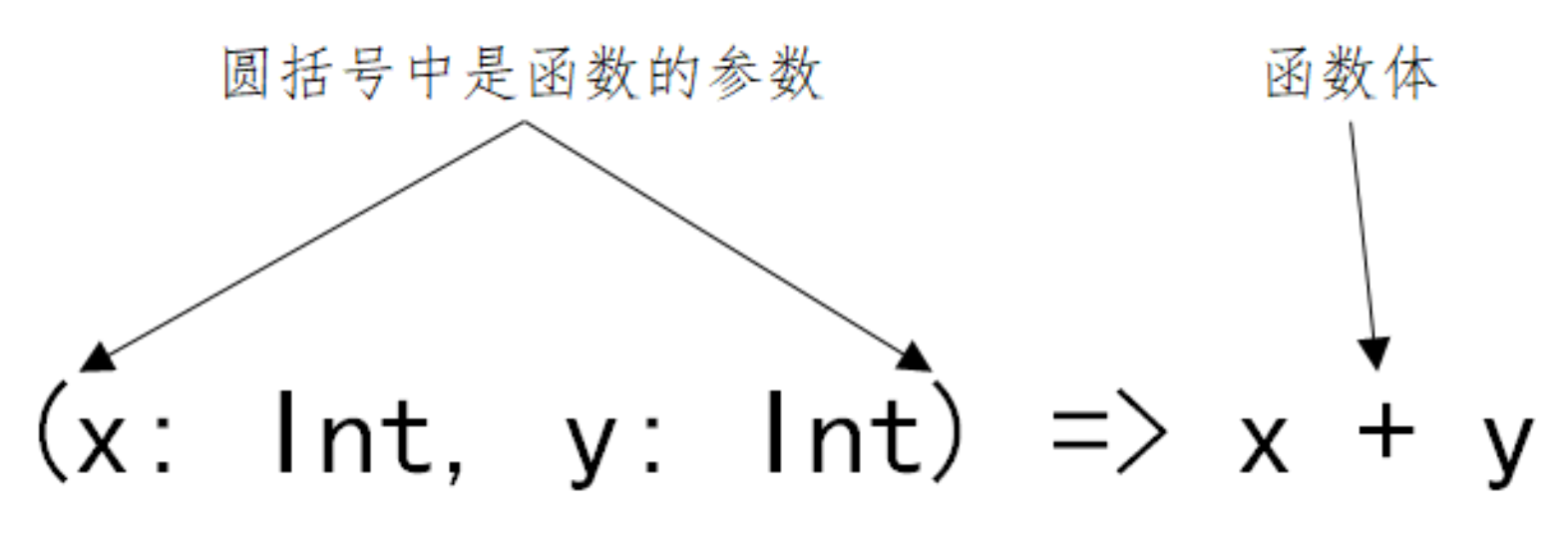把函数定义中的类型声明部分去除，剩下的就是函数的“值”，即函数字面量
在Scala中我们这样定义变量: `val 变量名: 类型 = 值` ;
我们可以用完全相同的方式定义函数:`val 函数名: 函数类型 = 函数字面量`

``````val add2: (Int, Int) => Int = (x, y) => x + y
// (Int, Int) => Int 为函数类型
// (x, y) => x + y 为函数的值
``````

在Scala中有自动类型推断，所以可以省略变量的类型 `val 变量名 = 值` 。 同样函数也可以这样:`val 函数名 = 函数字面量`

``````// 要让编译器进行自动类型推断，要告诉编译器足够的信息，所以添加了 x 的类型信息。
val add2 = (x: Int, y: Int) => x + y
``````

函数的定义:

``````val 函数名: (参数类型1，参数类型2) => (返回类型) = 函数字面量

val 函数名 = (参数1:类型1，参数2:类型2) => 函数体
``````

### 函数与方法的区别

``````// 方法
def addm(x: Int, y: Int): Int = x + y
// 函数
val addf = (x: Int, y: Int) => x + y
``````

Scala中的方法与函数有以下区别:

• Scala 中的方法与 Java 的类似，方法是组成类的一部分
• Scala 中的函数则是一个完整的对象。Scala 中用 22 个特质(从 Function1 到 Function22->不同参数长度)抽象出 了函数的概念
• Scala 中用 val 语句定义函数，def 语句定义方法
``````// 下面用三种方式定义了函数，其中第二种方式最常见
val adder1: (Int, Int) => Int = (x, y) => x+y
val adder2 = (x: Int, y: Int) => x+y

// Function2是特质，不能直接new
// new Function2[Int,Int,Int]{ ... } 其实是定义并实例化一个实现了 Function2 特质的类的 对象
val adder3 = new Function2[Int, Int, Int] {
override def apply(v1: Int, v2: Int): Int = {
v1 + v2
}
}
``````
• 方法不能作为单独的表达式而存在，而函数可以;
• 函数必须要有参数列表，而方法可以没有参数列表;
• 方法名是方法调用，而函数名只是代表函数对象本身;
• 在需要函数的地方，如果传递一个方法，会自动把方法转换为函数
``````// 方法不能作为单独的表达式而存在，而函数可以
scala> def addm(x: Int, y: Int): Int = x + y
def addm(x: Int, y: Int): Int

scala> val addf = (x: Int, y: Int) => x + y
val addf: (Int, Int) => Int = \$Lambda\$1154/250215657@1197b54e

^
error: missing argument list for method addm
Unapplied methods are only converted to functions when a function type is expected.

val res51: (Int, Int) => Int = \$Lambda\$1154/250215657@1197b54e

// 函数必须要有参数列表，而方法可以没有参数列表
scala> def m1 = "This is me"
def m1: String

scala> val f1 = () => "This is me"
val f1: () => String = \$Lambda\$1156/724595938@6358359e

// 方法名是方法调用
scala> m1
val res52: String = This is me

// 函数名代表函数对象
scala> f1
val res53: () => String = \$Lambda\$1156/724595938@6358359e

// 这才代表函数调用
scala> f1()
val res54: String = This is me

// 需要函数的地方，可以传递一个方法
scala> val list = (1 to 10).toList
val list: List[Int] = List(1, 2, 3, 4, 5, 6, 7, 8, 9, 10)

scala> def double(x: Int) = x*x
def double(x: Int): Int

scala> list.map(double(_))
val res55: List[Int] = List(1, 4, 9, 16, 25, 36, 49, 64, 81, 100)
``````

``````scala> def f1 = double _  //注意:方法名与下划线之间有一个空格
f1: Int => Int
scala> f1
res21: Int => Int = <function1>
``````

### 匿名函数与占位符

(参数名1: 类型1, 参数名2: 类型2, … …) => 函数体

``````// 将匿名函数作为参数传递给另一个函数
list.map((x: Int) => x + 1)

// x一定是Int类型，这里可以省略
list.map((x) => x + 1)

// 只有一个参数，小括号可以省略
list.map(x => x + 1)

// 使用占位符简化函数字面量
list.map(_ + 1)

// 实现将List中的每个元素*2 + 1，但是出错了
list.map(_ + _ + 1)
//<console>:13: error: missing parameter type for expanded function ((x\$1, x\$2) => x\$1.\$plus(x\$2).\$plus(1))

// 这样是可行的
list.map(2 * _ + 1)

// 通过reduce这个高阶函数，将list列表中的元素相加求和
list.reduce((x,y) => x + y)

// 使用占位符简化函数字面量
// 第一个下划线代表第一个参数，第二个下划线代表第二个参数
list.reduce(_ + _)
``````

• 第一个下划线代表第一个参数
• 第二个下划线代表第二个参数
• 第三个……，如此类推

### 高阶函数

``````object HighFunction {
def main(args: Array[String]): Unit = {

val func=(n) => "*" * n
// 把函数传入高阶函数
(1 to 5).map(func(_)).foreach(println)
/**
* *
* **
* ***
* ****
* *****
*/

// 定义一个高阶输出函数
val URLBuilder=(ssl: Boolean, domainName: String) => {
val schema = if (ssl) "https://" else "http://"

// 返回一个匿名函数
(endPoint: String, query: String) => s"\$schema\$domainName/\$endPoint?\$query"
}

val domainName="www.baidu.com"
def getUrl = URLBuilder(true, domainName)
val endpoint = "show"
val query = "id=1"
val url = getUrl(endpoint, query)
println(url)
// https://www.baidu.com/show?id=1
}
}

``````

### 闭包

``````// 普通的函数
val addMore1 = (x: Int) => x + 10
// 外部变量，也称为自由变量
var more = 10
// 闭包
val addMore2 = (x: Int) => x + more

more = 100
more = 1000
``````

1、闭包是一个函数
2、函数必须要有返回值
3、返回值依赖声明在函数外部的一个或多个变量，用Java的话说，就是返回值和定义的全局变量有关

### 柯里化

Scala中，柯里化函数的定义形式和普通函数类似，区别在于柯里化函数拥有多组参数列表，每组参数 用小括号括起来。
Scala API中很多函数都是柯里化的形式。

``````// 使用普通的方式
def add1(x: Int, y: Int) = x + y

// 使用闭包的方式，将其中一个函数作为返回值
def add2(x: Int) = (y:Int) => x + y

// 使用柯里化的方式
def add(x: Int)(y: Int) = x + y

val res61: Int => (Int => Int)
val res62: Int => (Int => Int)
//在这个函数中，接收一个x为参数，返回一个匿名函数，这个匿名函数的定义是:接收一个Int型参数y，函 数体是x+y。
``````

### 部分应用函数

``````// 定义一个函数
def add(x:Int, y:Int, z:Int) = x+y+z

// Int不能省略

// 省略了全部的参数，下面两个等价。第二个更常用
``````

### 偏函数

Scala中的Partial Function是一个trait，其类型为PartialFunction[A,B]，表示:接收一个类型为A的参 数，返回一个类型为B的结果。

``````// 过滤List中的String类型的元素，并将Int类型的元素加1。
object PartialFunctionDemo {
def main(args: Array[String]): Unit = {

val partialFunction = new PartialFunction[Any, Int] {
override def isDefinedAt(x: Any): Boolean = {
println(x.toString)
x.isInstanceOf[Int]
}

override def apply(v1: Any): Int = v1.asInstanceOf[Int]+1
}

// 等价于上面
val partialFunction2: PartialFunction[Any, Int] = {case x: Int => x+1}

val list = List("A", 2, "ff", 34, "fdf", 12)

list.collect(partialFunction).foreach(println)

// 等价于上一行
list.collect{case x: Int => x+1}.foreach(println)
}
}

``````

## 集合

### 可变和不可变集合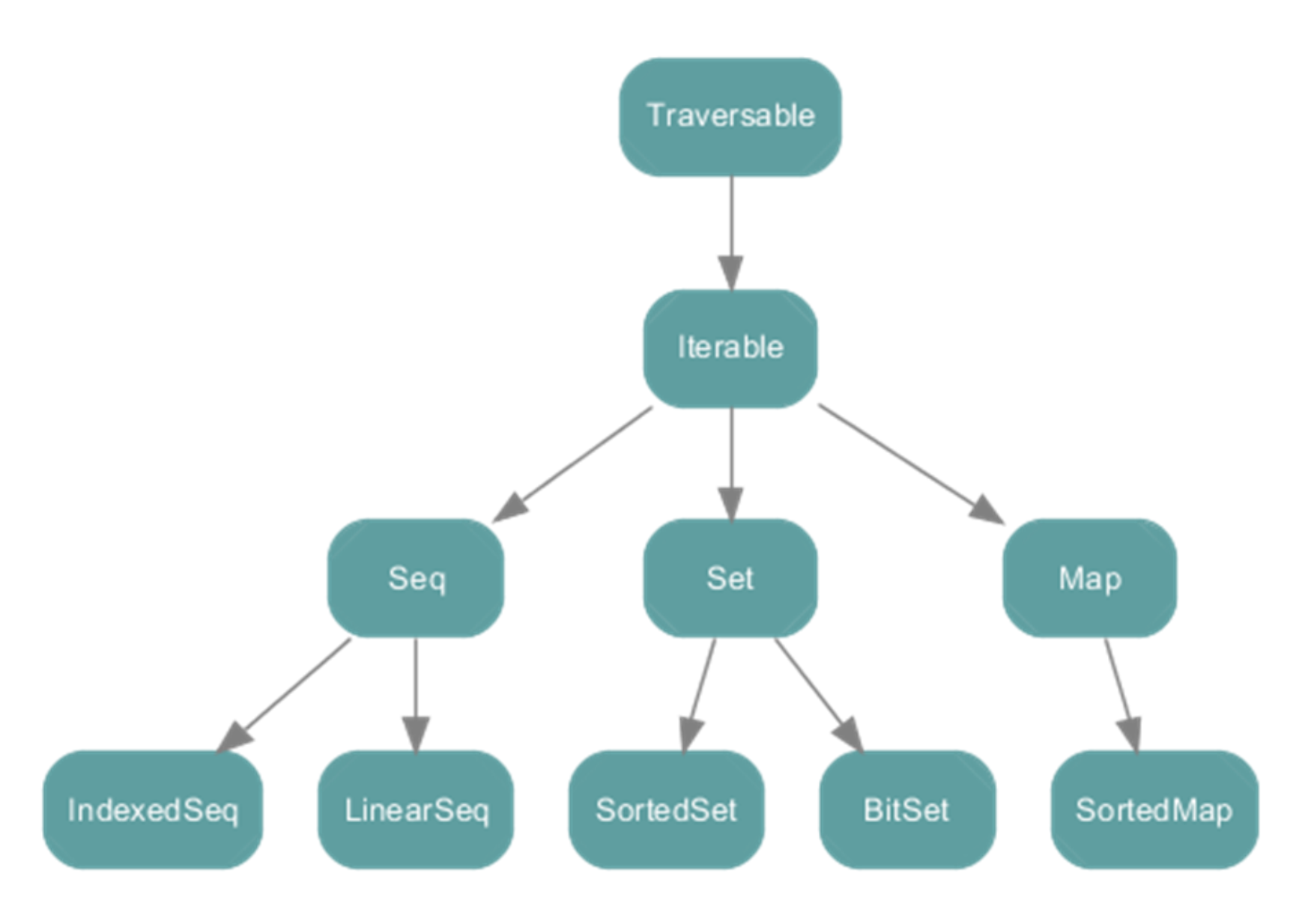• 不可变集合：是指集合内的元素一旦初始化完成就不可再进行更改，任何对集合的改变都将生成一个新的 集合，scala.collection.immutable
• 可变集合：提供了改变集合内元素的方法，scala.collection.mutable;

Scala集合有三大类:Seq(序列)、Set(集)、Map(映射); 所有的集合都扩展自Iterable特质。

• immutable不可变集合• mutable可变集合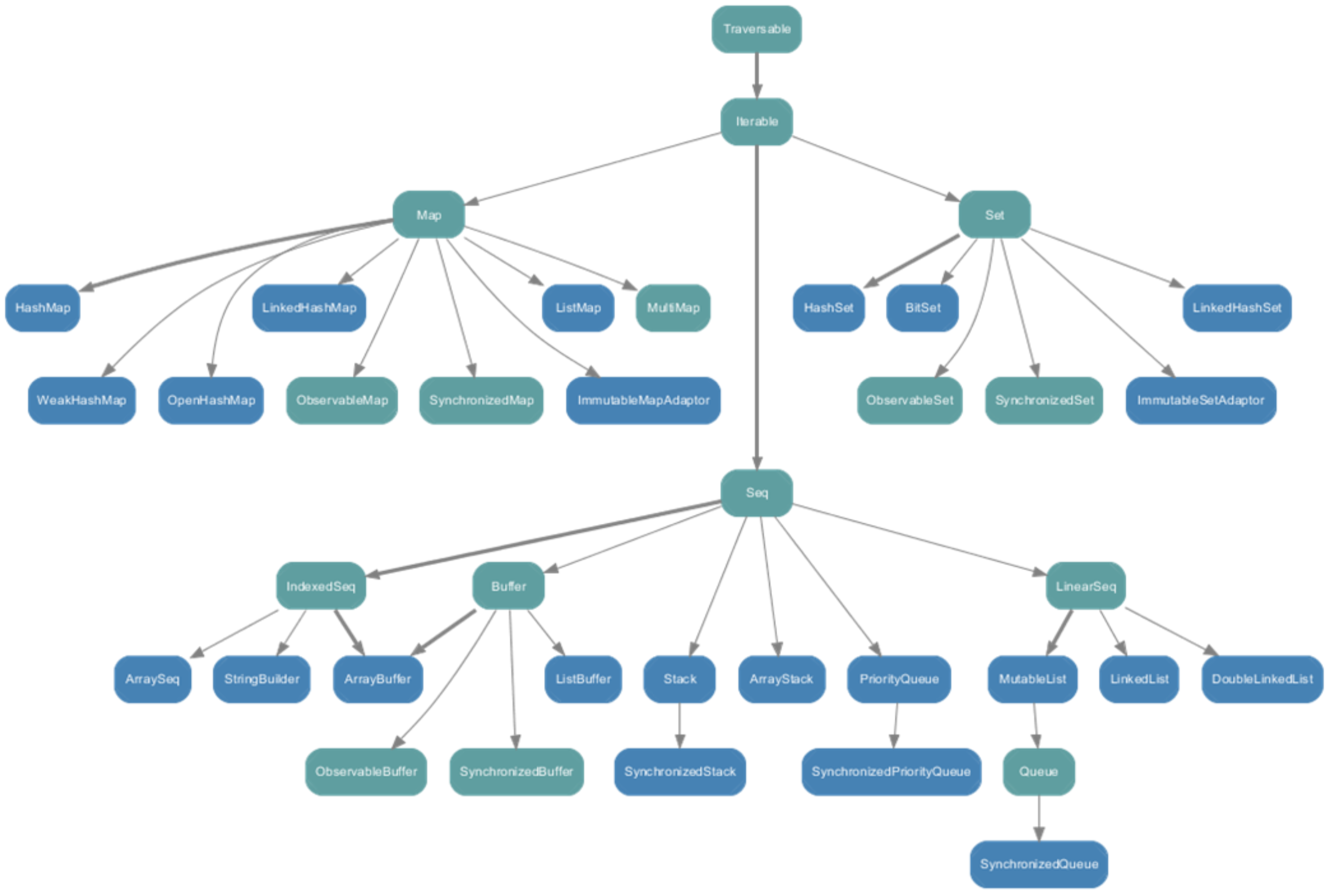• String属于IndexedSeq
• Queue队列和Stack堆这两个经典的数据结构属于LinearSeq
• Map体系下有一个SortedMap，说明Scala中的Map是可以支持排序的 * mutable可变集合中Seq中的Buffer下有ListBuffer，它相当于可变的List列表;
• List列表属于Seq中的LinearSeq

### Seq

Seq代表按照一定顺序排列的元素序列;

Seq提供了两个重要的子特质:

• IndexedSeq:提供了快速随机访问元素的功能，它通过索引来查找和定位的

• List List代表元素顺序固定的不可变的链表，它是Seq的子类，在Scala编程中经常使用。 List是函数式编程语言中典型的数据结构，与数组类似，可索引、存放类型相同的元素。 List一旦被定义，其值就不能改变。
• tail返回的是除第一个元素外的其它元素构成的新列表

Scala定义了一个空列表对象Nil，定义为List[Nothing]
借助 Nil 可将多个元素用操作符 :: 添加到列表头部，常用来初始化列表;
操作符 ::: 用于拼接两个列表;

``````object ListDemo {
def main(args: Array[String]): Unit = {

// :: 是右结合的
val list1 = 1 :: 2 :: 3 :: 4 :: Nil
val list2 = 5 :: 6 :: 7 :: 8 :: Nil

// 使用 ::: 拼接List
val list3 = list1 ::: list2

println(list3.last)   // 最后一个元素
println(list3.init)   // 去掉最后一个元素的集合
println(list3.tail)   // 去掉第一个元素的集合

val list4 = List(3, 1, 2, 5, 6)
println(quickSort(list4))
}

// 实现快速排序
def quickSort(list: List[Int]):List[Int] = {
list match {
case Nil => Nil
// partition 根据条件把数组分割成两部分
case head :: tail => val (less, greater) = tail.partition(_ < head)
}
}
}
``````
• Queue 队列Queue是一个先进先出的结构。
队列是一个有序列表，在底层可以用数组或链表来实现。
先进先出的原则，就是先存入的数据，要先取出，后存入的数据后取出。
在Scala中，有scala.collection.mutable.Queue和scala.collection.immutable.Queue,一般来说，我 们使用的是scala.collection.mutable.Queue

``````object QueueDemo {
def main(args: Array[String]): Unit = {
// 使用可变的queue
val queue1 = new mutable.Queue[Int]()

println(queue1)

queue1 += 1
queue1 ++= List(2, 3, 4)
println(queue1)

val dequeue = queue1.dequeue()
println(dequeue)
println(queue1)

queue1.enqueue(5, 6, 7)

println(queue1)

println(queue1.last)

}
}

``````

### Set

Set(集合)是没有重复元素的对象集合，Set中的元素是唯一的; Set分为可变的和不可变的集合;

``````object SetDemo {
def main(args: Array[String]): Unit = {
val set = Set(1, 2, 3, 4, 5, 6)
// drop 无效
set.drop(2)
println(set)

import scala.collection.mutable.Set
val mutableSet = Set(3, 4, 5)
// 添加，移除
mutableSet.remove(5)
println(mutableSet)

// 使用 += -= 添加，移除元素
mutableSet += 10
mutableSet -= 4
println(mutableSet)

// & intersect 求交集
println("=================intersect====================")
println(Set(1, 2, 3) & Set(2, 3, 4))
println(Set(1, 2, 3) intersect Set(2, 3, 4))

// ｜ ++ union 求合集
println("=================union====================")
println(Set(1, 2, 3) | Set(2, 3, 4))
println(Set(1, 2, 3) ++ Set(2, 3, 4))
println(Set(1, 2, 3) union Set(2, 3, 4))

// &～ -- diff 求差集，A中有-B中有的=A中独有的
println("=================diff====================")
println(Set(1, 2, 3) &~ Set(2, 3, 4))
println(Set(1, 2, 3) -- Set(2, 3, 4))
println(Set(1, 2, 3) diff Set(2, 3, 4))

// A & B 独有的元素
println((Set(1, 2, 3) | Set(2, 3, 4)) -- (Set(1, 2, 3) & Set(2, 3, 4)))
}
}

``````

### Map

Map(映射)是一系列键值对的容器;
Scala 提供了可变的和不可变的两种版本的Map， 分别定义在包 scala.collection.mutable 和 scala.collection.immutable 里;

``````object MapDemo {
def main(args: Array[String]): Unit = {
// 两种定义map的方式
val map1 = Map('a'-> 1, 'b' -> 2)
val map2 = Map(('a', 1), ('b', 2))

map1.keys.foreach(println)
map1.values.foreach(println)

println(map1('b'))
//    println(map1('c'))

// get 返回一个option对象，不存在就返回None， 存在就Some
println(map2.get('c'))

if (map2.get('b') != None) {
println("Some")
} else {
println("None")
}

// key不存在就返回默认值
val num2 = map1.getOrElse('d', 0)
println(num2)

// 可变的map
val map3 = collection.mutable.Map("a"->1, "b"->2)
println(map3)
map3("a") = 10
println(map3)
map3("c")=20
println(map3)

// 使用 += -= 添加删除元素
map3 += ("d"->4, "f"->5)
println(map3)

map3 -= "d"
println(map3)

// exchange key & value
val kv: collection.mutable.Map[Int, String] = for ((k, v) <- map3) yield (v, k)
println(kv)
map3.map(x=>(x._2,x._1)).foreach(println)

// 使用zip的方式构建map
val a = List(1, 2, 3)
val b = List("a", "b", "c")
val c = a.zip(b).toMap   //返回类型是 immutable.Map
println(c)
println(map3.mapValues(_+10))
}
}
``````

### 集合常用算子

• map、foreach & mapValues
• foreach无返回值(准确说返回void)，用于遍历集合
• map返回集合对象，用于将一个集合转换成另一个集合
• 操作 Map集合时，mapValues用于遍历value，是map操作的一种简化形式;
``````// Range(20, 0, -2)用给定的步长值设定一个范围，从开始到结束(不包含)。
//Map(20 -> 0,18 -> 1,16 -> 2,14 -> 3,12 -> 4,10 -> 5,8 -> 6,6 -> 7,4 -> 8,2 -> 9)

val map = Range(20, 0, -2).zipWithIndex.toMap

// 将map集合中的value值+100
map.map(elem => (elem._1, elem._2 + 100))
map.map{case (k,v) => (k, v+100)}
// mapValues的表达最简洁 map.mapValues(_+100)
``````
• flatten & flatMap
• flatten的作用是把嵌套的结构展开，把结果放到一个集合中;
• 在 flatMap 中传入一个函数，该函数对每个输入都返回一个集合(而不是一个元素)，最后把生成的多 个集合“拍扁”成为一个集合;
• flatMap = flatten + map 或 flatMap = map + flatten (如果需要处理的数组只有一层结构，就先map，然后flatten)
• collect collect通过执行一个并行计算(偏函数)，得到一个新的数组对象

``````object CollectDemo {
//通过下面的偏函数，把chars数组的小写a转换为大写的A
val fun: PartialFunction[Char, Char] = {
case 'a' => 'A'
case x => x
}
def main(args: Array[String]): Unit = {
val chars = Array('a', 'b', 'c')
val newchars = chars.collect(fun)
println("newchars:" + newchars.mkString(","))
}
}
``````
• reduce reduce可以对集合当中的元素进行归约操作;
还有 reduceLeft(reduce) 和 reduceRight ，reduceLeft 从左向右归约，reduceRight 从右向左归约;

``````val lst1 = (1 to 10).toList
lst1.reduce(_+_)

// 我们说过一个占位符代表一个参数，那么两个占位符就代表两个参数。根据这个思路改写等价的语句
// x类似于buffer，缓存每次操作的数据;y每次操作传递新的集合元素
lst1.reduce((x, y) => x + y)

// 利用reduce操作，查找 lst1 中的最大值

lst1.reduce((x,y) => if (x>y) x else y)
// reduceLeft、reduceRight lst1.reduceLeft((x,y) => if (x>y) x else y)
lst1.reduceRight((x,y) => if (x>y) x else y)
``````
• sorted sortwith & sortby

### 与Java集合的转换

``````import scala.collection.JavaConverters._
val list: Java.util.List[Int] = List(1,2,3,4).asJava
val buffer: scala.collection.mutable.Buffer[Int] = list.asScala
``````

## 隐式机制

### 隐式转换

Scala会根据隐式转换函数的签名，在程序中使用到隐式转换函数接收的参数类型定义的对象时，会自

• 首先得有一个隐式转换函数
• 使用到隐式转换函数接收的参数类型定义的对象
• Scala自动传入隐式转换函数，并完成对象的类型转换

• implicit关键字只能用来修饰方法、变量、参数
• 隐式转换的函数只在当前范围内才有效。如果隐式转换不在当前范围内定义，那么必须通过 import语句将其导入

### 隐式转换函数

Scala的隐式转换最核心的就是定义隐式转换函数，即implicit conversion function。 定义的隐式转换函数，只要在编写的程序内引入，就会被Scala自动使用。 隐式转换函数由Scala自动调用，通常建议将隐式转换函数的名称命名为“one2one”的形式。

``````class Num
class RichNum(num: Num) {
def rich() : Unit = {
println("Hello implicit !")
}
}

object ImplicitDemo {
implicit def num2RichNum(num: Num):RichNum = new RichNum(num)

def main(args: Array[String]): Unit = {
val num = new Num

// num对象并没有rich方法，编译器会查找当前范围内是否有可转换的函数
// 如果没有则编译失败，如果有则会调用。
num.rich()
}
}
``````

``````class SpecialPerson(var name: String)
class Older(var name: String)
class Student(var name: String)
class Worker(var name: String)

object ImplicitDemoTwo {

def buySpecialTicketWindow(person: SpecialPerson): Unit = {
if (person == null) {
println("You are not special person!!")
} else {
println(person.name + " Here's your ticket!")
}
}

implicit def any2SpecialPerson(any: Any):SpecialPerson = {
any match {
case any: Older => new SpecialPerson(any.asInstanceOf[Older].name)
case any: Student => new SpecialPerson(any.asInstanceOf[Student].name)
case _ => null
}
}

def main(args: Array[String]): Unit = {
val stu = new Student("stu")
val older = new Older("old man")
val worker = new Worker("Worker")

}
}
``````

### 隐式参数和隐式值

• 当前作用域内可见的val或var定义隐式变量
• 隐式参数类型的伴生对象内隐式值
``````object DoublelyDemo {
//在print函数中定义一个隐式参数fmt
def print(num: Double)(implicit fmt: String): Unit = {
println(fmt format(num))
}

def main(args: Array[String]): Unit = {

print(3.1415)("%.1f")

//定义一个隐式变量
implicit val printFmt = "%.3f"
//当调用print函数时没有给第二个隐式参数赋值，
//那么Scala会在当前作用域内寻找可见的val或var定义的隐式变量，一旦找到就会应用
print(3.1415)
}
}
``````

## 类型参数

Scala的类型参数与Java的泛型是一样的，可以在集合、类、函数中定义类型参数，从而保证程序更好的

### 泛型类

``````class Stack[T1, T2, T3](name: T1) {
var age: T2 = _

def getInfo:Unit = {
}
}

object GenericDemo {
def main(args: Array[String]): Unit = {
val stack = new Stack[String, Int, String]("lisi")
stack.age = 20
stack.getInfo
}
}
``````

### 泛型函数

``````object GenericityFunction {
def getCard[T](content: T) = {
content match {
case content: Int => s"card:\$content is Int "
case content: String => s"card:\$content is String"
case _ => s"card:\$content"
}
}
def main(args: Array[String]): Unit = {
println(getCard[String]("hello"))
println(getCard(1001))
}
}
``````

### 协变和逆变

Scala的协变和逆变是非常有特色的，完全解决了Java中的泛型的一大缺憾!

• 协变定义形式如:trait List[+T] {} : 当前类及其子类
• 逆变定义形式如:trait List[-T] {} : 当前类及其父类
``````class Master
class Professor extends Master
class Teacher

//这个是协变，当前类及其子类
//class Card[+T]

//逆变, 当前类及其父类
class Card[-T]

object CovarianceDemo {
def enterMeet(card: Card[Professor]): Unit = {
println("Welcome !")
}

def main(args: Array[String]): Unit = {
val masterCard = new Card[Master]
val professorCard = new Card[Professor]
val teacher = new Card[Teacher]

enterMeet(masterCard)
enterMeet(professorCard)
//    enterMeet(teacher)
}
}
``````

## Akka

Akka是Java虚拟机平台上构建高并发、分布式和容错应用的工具包和运行时。 Akka用Scala语言编写，同时提供了Scala和Java的开发接口。 Akka处理并发的方法基于Actor模型，Actor之间通信的唯一机制就是消息传递。

### Actor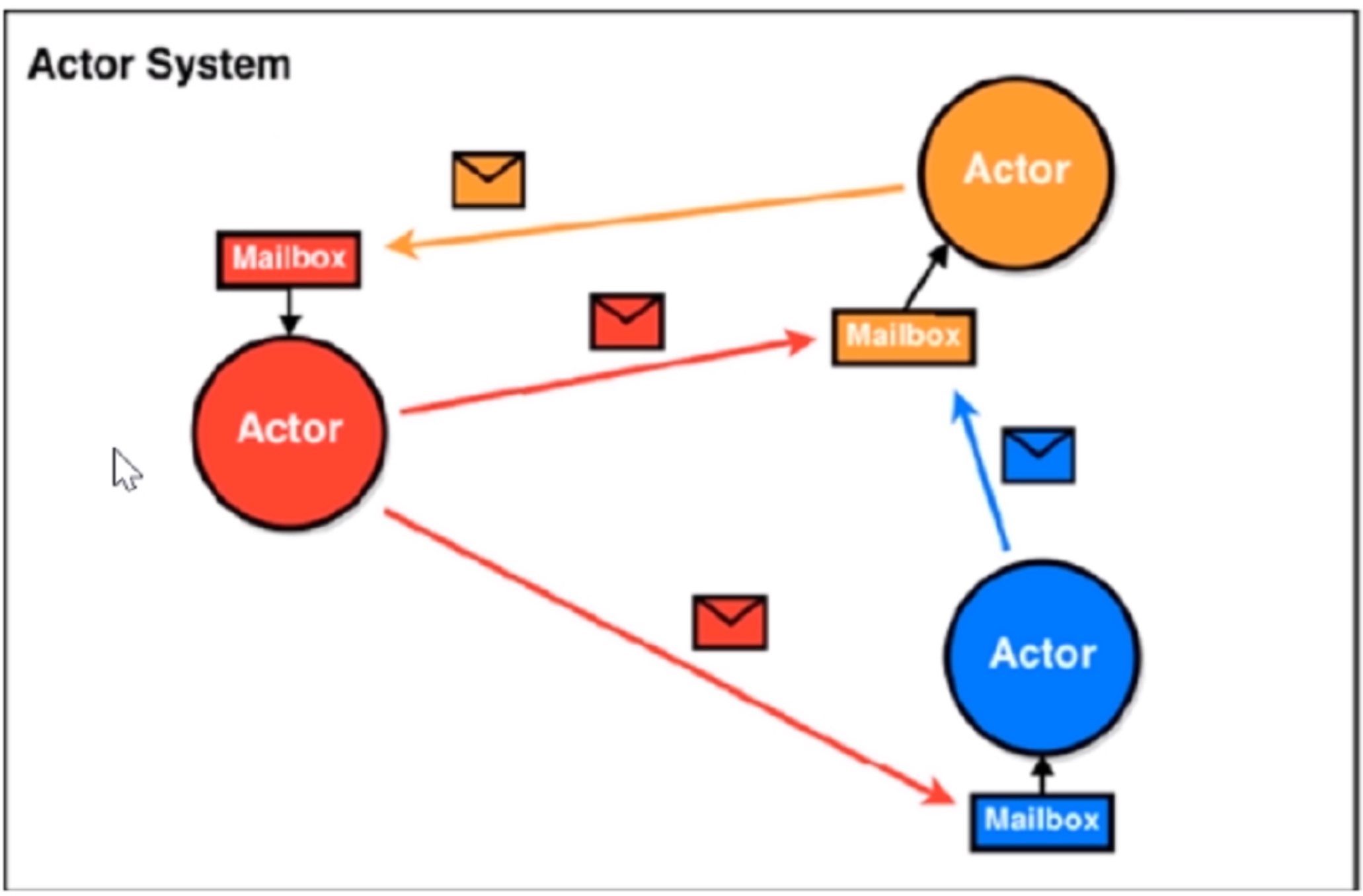Scala的Actor类似于Java中的多线程编程。 但是不同的是，Scala的Actor提供的模型与多线程有所不同。Scala的Actor尽可能地避免锁和共享状态，从而避免多线程并发时出现资源争用的情况，进而提升多线程编程的性能。 Actor可以看作是一个个独立的实体，Actor之间可以通过交换消息的方式进行通信，每个Actor都有自己的收件箱(Mailbox)。

### Akka案例

• maven依赖
``````<!-- 定义一下常量-->
<properties>
<encoding>UTF-8</encoding>
<scala.version>2.12.3</scala.version>
<scala.compat.version>2.11</scala.compat.version>
<akka.version>2.4.17</akka.version>
</properties>

<dependencies>
<!-- 添加akka的actor依赖 -->
<dependency>
<groupId>org.scala-lang</groupId>
<artifactId>scala-actors</artifactId>
<version>2.11.8</version>
</dependency>
<!-- https://mvnrepository.com/artifact/com.typesafe.akka/akka-actor -->
<dependency>
<groupId>com.typesafe.akka</groupId>
<artifactId>akka-actor_2.11</artifactId>
<version>2.3.16</version>
</dependency>

<!-- 添加akka的actor依赖 -->
<dependency>
<groupId>com.typesafe.akka</groupId>
<artifactId>akka-actor_\${scala.compat.version}</artifactId>
<version>\${akka.version}</version>
</dependency>
<!-- 多进程之间的Actor通信 -->
<!-- https://mvnrepository.com/artifact/com.typesafe.akka/akka-remote -->
<dependency>
<groupId>com.typesafe.akka</groupId>
<artifactId>akka-remote_\${scala.compat.version}</artifactId>
<version>\${akka.version}</version>
</dependency>
</dependencies>
``````
• 代码

``````import scala.io.StdIn

class HelloActor extends Actor {

// 接收消息并处理
case "eat" => println("eat a banana")
case "sleep" => println("good night")
case "bye" => {
//关闭自己
context.stop(self)
//关闭ActorSystem
context.system.terminate()
}
}
}

object HelloActor {
//创建线程池对象MyFactor，myFactory为线程池的名称
private val MyFactory: ActorSystem = ActorSystem("MyFactory")

// 通过MyFactory.actorOf方法来创建一个actor;
private val helloActorRef: ActorRef = MyFactory.actorOf(Props[HelloActor], "helloActor")

def main(args: Array[String]): Unit = {
var flag = true
while (flag) {
println("say something: ")